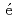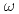# Numerical simulation of sodium mist behavior in turbulent Rayleigh-Bnard convection using new developed mist models
In order to evaluate the mist behavior in the cover gas region of Sodium-cooled Fast Reactors (SFRs) in good accuracy, turbulent model for Rayleigh-Bnard convection (RBC) was selected, and the Reynolds-averaged number density and momentum equations for mist behavior were developed and incorporated into the OpenFOAM code. In the first stage, the RBC in a simple parallel channel was calculated using Favre-averaged k-SST model. The average temperature and flow characteristics agreed well with results from DNS, LES, and experiments. Then the basic heat transfer experiment simulating the cover gas region of SFRs was calculated using this turbulent model and new mist models. The calculated average temperature distribution in the height direction and the mist mass concentration agreed well with the experimental results. We developed a method that could simulate the mist behavior in turbulent RBC environments and the cover gas region of SFRs with high accuracy.